×
Log in to StudySoup
Get Full Access to ASU - CHM 101 - Study Guide - Midterm
Join StudySoup for FREE
Get Full Access to ASU - CHM 101 - Study Guide - Midterm

Already have an account? Login here
×
Reset your password

# chm 101 Description

##### Uploaded: 02/24/2017
8 Pages 169 Views 0 Unlocks
Reviews

CHM 101 Exam 1 Review Questions Key Answers to Multiple Choice 1. 10:45am Section: Answer is C11:50am Section: Answer is D 2. A 3. D 4.E (Molecules consist of 2 or more connected atoms, and a pure substance is an element or a compound.) 5. D 6. E 7. E 8. C 9. A 10. D 11. E 12. E 13. B 14. B A (Cl2 15. is the element form of chlorine)(The ion form is Cl) 16. D 17. D 18. E 19. C 20. A 21. D

## How is the compound you chose classified?. 23. B 24. C 25. C 26. E

27. A (a mixture of bromine and water)

CHM 101 P. M Marks  Exam 1 Ex xam Prep Q Questions  Instructions : The purpos se of this activ vity is to give e you practice e with multipl le-choice que stions.  ∙ Answ wer the multip ple-choice qu uestions. Circl le your answe er choice for e each.  ∙ Chec ck answers wi ith others and d with instruct tors.  ∙ In cla ass today, foc cus on answer ring the follow wing multiple e-choice ques stions. Circle e the best answ wer.  ∙ An a answer sheet w will be distrib buted 10 minu utes before the e end of class s.  ∙ If yo u have time a after answerin ng all the mul ltiple-choice q questions, go  back and ans swer the follo ow-up  quest tion that com mes after the la ast answer cho oice.  ∙ The e equations/info formation and d the periodic  1. What room table provide ed with exam  1 are on the l last two page s. m should I go  to on Friday, , February 3,  at my regular rly scheduled  d class time?  A. LIB  B. LIB  L60 at 10:45a am  L62 at 11:50a am  C. Schw wada 210 at 1 D. PS H 0:45am  H-151 at 11:50 0am  E. The e exam is onlin ne.  S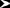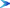Should I bring g my ID, calc culator, and pe encils? Shou uld I arrive a l little early?  2. Atoms that t are isotopes s of the same e element diffe er in ________ ________.  A. number o A B. number o B C. number o C D. charge  D f neutrons  f protons  f electrons  E. atomic nu E umber For each inco Frrect answer,  identify a pa ir of substanc ces that would d differ in tha at way.  3. Which of t the following A. The nucle statements ab bout atoms, io ons, and suba atomic particl les is true?  A eus of an atom m has no mass s.  B. Neutrons  B are negativel ly charged.  C. Electrons  C D. The nucle exist in the n nucleus.  most of the m D eus makes up  mass of an ato om.  E. A cation h E has more elec ctrons than pr otons.  Modify each iM incorrect state ement to mak ke it correct.  4. Which of  the following g is a pure sub bstance that is s composed o of molecules? ?  I.   II.   III.    IV.   V.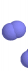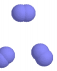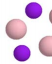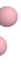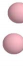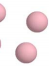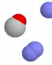## What is the likely identity of the metal?## What is the formula for this ion?If you want to learn more check out where are the longest muscle fibers found in the body?
We also discuss several other topics like econ 100a midterm
If you want to learn more check out plant physiology exam
If you want to learn more check out math 205 concordia
Don't forget about the age old question of comm107 umd
We also discuss several other topics like cognitive psychology exam 1 study guide

A. V V B. I II  C. I III  Classify each  CD. IV V  E. I a and V  as an elemen nt, compound, , or mixture. 5. Which of the following is NOT composed of ions?  A. CaO  B. FeO  C. MgO  D. CO  E. All of these are composed of ions.  How is the compound you chose classified?  6. A monatomic ion with a 3+ charge has the same number of electrons as an argon atom. What is the formula  for this ion?  A. Al3+ B. N3+ C. P3+ D. Ar3+ E. Sc3+ Which of these has the same number of electrons as a noble gas other than argon?  7. Which of the following has the greatest number of electrons?  A. Ca2+ B. K+ C. Cl− D. S2− E. These all have the same number of electrons.  Which has the greater number of protons?  8. A rectangular block of an unknown metal with a mass of 456 g is measured to have a length, width, and height  of 10.2 cm, 5.08 cm, and 3.26 cm, respectively. What is the likely identity of the metal?  A. zinc, density = 7.14 g/mL  B. lead, density = 11.3 g/mL  C. aluminum, density = 2.70 g/mL  D. nickel, density = 8.91 g/mL  E. titanium, density = 4.51 g/mL  What would be the masses of the same size blocks of the other metals?  9. Which of the following statements is correct?  A. Natural gas burning to produce water and carbon dioxide is an example of a chemical change.  B. In a physical change, atoms rearrange to form new substances.  C. When a substance undergoes a chemical change, its chemical composition does not change.  D. Formation of rust on an old car is an example of a physical change.  E. The boiling point of nitrogen is −196°C. At −210°C, nitrogen is expected to be a gas.  Modify each incorrect statement to make it a true statement.  10. Identify the number of protons and neutrons in an atom of the isotope 64Zn.  A. 64 protons, 34 neutrons  B. 64 protons, 30 neutrons  C. 30 protons, 30 neutrons  D. 30 protons, 34 neutrons  E. More information is needed.  How many electrons does an atom zinc-64 have?   11. Naturally-occurring gallium (Ga) is made of two isotopes: gallium-69 and gallium-71. Its relative atomic  mass is given on the periodic table. Which of the following statements is true?  A. Gallium’s relative atomic mass is 70.00 amu.  B. Both isotopes have the same mass: 69.72 amu.  C. The isotopes are present in the same percentages (50% and 50%).  D. Gallium-71 is present in the larger percent abundance.  E. Gallium-69 is present in the larger percent abundance.  Is relative atomic mass a weighted average?  12. Which of the following has a positive charge?  A. a sodium atom  B. a sodium ion  C. a proton  D. an electron  E. both a sodium ion and a proton  For those that do not have a positive charge, identify whether each has a negative charge or no  charge.  13. Which of the following calculated results should have two significant figures?  A. 2.0 cm + 11.1 cm  B. 5.5 cm – 2.1 cm  C. 5.25 cm × 9.10 cm  D. 0.050 cm × 0.009 cm  E. 10.5 cm × 15.8 cm  Determine the number of significant figures in the calculated results that do not have two significant  figures.  14. How many significant figures are in the measurement 0.002502 L?  A. 3  B. 4  C. 5  D. 6  E. 7  What is the value converted to mL? Does it have the same number of significant figures?  15. Chlorine gas is a yellow-green gas that is highly toxic. What is the formula for chlorine gas?  A. Cl2 B. Cl2−  C. Cl22−  D. Cl− E. Cl  Which of these is the correct formula for chloride ion?  16. Which of the following statements is correct?  A. Molecular compounds consist of two or more metal elements.  B. Molecular compounds are generally strong electrolytes in aqueous solution.  C. Ionic compounds generally consist of metal cations and metal anions.  D. Ionic compounds generally dissociate into ions when dissolved in water.  E. Acids are nonelectrolytes.  Modify each incorrect statement to make it a true statement.   17. Which of the following is the correct IUPAC name of the compound with the formula P2O5?  A. potassium oxide  B. dipotassium pentoxide  C. phosphorus oxide  D. diphosphorus pentoxide  E. phosphorus(V) oxide  Which of the others are correct IUPAC names for a compound?  18. What is the formula for a compound composed of aluminum ions and nitrate ions?  A. AlN  B. Al3N  C. Al(NO3)2 D. AlNO2 E. Al(NO3)3 Which is the formula for aluminum nitride?  19. Elements in which group always form monatomic ions with a negative 1 (1−) charge?  A. alkali metals  B. alkaline earth metals  C. halogens  D. noble gases  E. transition metals  For each incorrect group that contains elements that form ions with the same charge, identify the  charge those elements would have when they form ions.  20. Which of the following elements is classified as a nonmetal in period 3?  A. phosphorus  B. potassium  C. bromine  D. germanium  E. krypton  For each incorrect answer, identify the element as a metal, nonmetal, or metalloid.  21. What is the correct formula for aluminum sulfate?  A. AlSO3  B. AlSO4  C. Al2S3  D. Al2(SO4)3  E. Al3(SO4)2  Which of these is the correct formula for aluminum sulfide?  22. Which of the following compounds contains a metal ion with a charge of 3+?  A. Cu3PO4  B. Cr(NO3)2  C. Mn2O3  D. PbO2  E. Fe3(PO4)2  Give the correct IUPAC name for each compound.  23. What is the correct IUPAC name for CoCl3?  A. cobalt chloride  B. cobalt(III) chloride  C. cobalt(IV) chloride  D. cobalt trichloride  E. cobalt(I) chloride  What is the charge on the cobalt ion in this compound? 24. In which case does the formula of the acid NOT match the acid name?  A. hydrosulfuric acid – H2S(aq)  B. sulfuric acid – H2SO4(aq)  C. chloric acid – HCl(aq)  D. carbonic acid – H2CO3(aq)  E. nitric acid – HNO3(aq)  For the answer you chose, write the correct formula for the name give, and then write the correct  name for the formula given.  25. Which of the following has the largest mass? (Hint: Convert all to gram units)  A. 4.1 × 10−4 kg  B. 1.8 × 104 mg  C. 25 g  D. 6.5 × 106 μg  E. 7.6 × 10−1 g  Arrange these quantities in order of increasing mass.  26. A bicyclist is traveling at 6.7 meters per second. What is her speed in units of kilometers per hour?  (1 min = 60 sec and 1 hr = 60 min)  A. 0.40 km/hr  B. 1.9 km/hr  C. 111 km/hr  D. 2.4×104 km/hr  E. 24 km/hr  What is the bicyclist’s speed in miles per hour? (1 km = 0.621 mi)  27. Which of the following represents a mixture?  A. Br2(aq)  B. CH3OH(l)  C. NaCl(s)  D. O2(g)  E. none of these  Classify each of the others as a pure element or pure compound.
Page ExpiredIt looks like your free minutes have expired! Lucky for you we have all the content you need, just sign up here